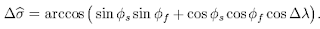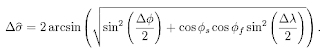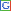## Monday, April 12, 2010

### Microsoft Access Tips & Tricks: Great Circle Distance

The great circle distance or orthodromic distance is the shortest distance between any two points on the surface of a sphere measured along a path on the surface of the sphere (as opposed to going through the sphere's interior). Because spherical geometry is rather different from ordinary Euclidean geometry, the equations for distance take on a different form. The distance between two points in Euclidean space is the length of a straight line from one point to the other. On the sphere, however, there are no straight lines. In non-Euclidean geometry, straight lines are replaced with geodesics. Geodesics on the sphere are the great circles (circles on the sphere whose centers are coincident with the center of the sphere).

If you are interested, you can find my earlier posts on finding the median, the mode, the geometric and harmonic means, ranking every row in a query, selecting random rows out of a table, calculating running sums and averages, calculating running differences, creating histograms, calculating probability masses out of given data, calculating cumulative distributions out of given data, finding percentile scores, percentile values, calculating distinct counts, full outer joins, parameter queries, crosstab queries, working with system objects, listing table fields, finding unmatched rows, calculating statistics with grouping, job-candidate matching, and job-candidate matching with skill levels.

Between any two points on a sphere which are not directly opposite each other, there is a unique great circle. The two points separate the great circle into two arcs. The length of the shorter arc is the great circle distance between the points.

Between two points which are directly opposite each other, called antipodal points, there are infinitely many great circles, but all great circle arcs between antipodal points have the same length, i.e. half the circumference of the circle, or πr, where r is the radius of the sphere.

Great circle distances are very useful for finding the shortest distances between points on a sphere. Since the shape of the earth can be approximated to be a sphere with negligible loss of accuracy, the distance between different points on the earth can be calculated to a great degree of accuracy using great circle distance formulae.

If we have a database that has a table that lists the coordinates of various points on the earth (coordinates of cities, airports, etc.), then we may want to use these formulae to calculate the great circle distance between them at some point. For the purpose of this post, we will assume that our database contains coordinates in the form of degrees, minutes and seconds for both latitude and longitude.

We will also assume that latitude values can go from -90 to +90 degrees, with latitudes of points on the northern hemisphere of the earth being positive and latitudes of points on the southern hemisphere being negative. All points on the equator will have a latitude of 0, while the north pole will have a latitude of +90 and the south pole would have a latitude of -90.

Similarly, we will assume that longitudes range from -180 degrees to +180 degrees. Points on the prime meridian that passes through Greenwich, England, would have a longitude of 0, while points on the opposite side of the earth from that line would have a longitude of either +180 or -180 (the +180 and -180 lines of longitude coincide on top of each other). We will assume that points in the western hemisphere have negative longitudes while points in the eastern hemisphere have positive longitudes.

Now, that we have established the basics of the table structure and conventions regarding signs and quantities, we can look at the formulae used for computing the great circle distance between two points. Let$\phi_s,\lambda_s;\ \phi_f,\lambda_f\;\!$ be the coordinates (latitude, longitude) of the start point and final point respectively. Let$\Delta\phi,\Delta\lambda\;\!$ be the differences in latitude and longitude respectively between the two points. The distance between them is calculated in terms of$\Delta\widehat{\sigma}\;\!$, which represents the angular distance between the points subtended at the center of the sphere. The actual distance between the points is r*$\Delta\widehat{\sigma}\;\!$, where r is the radius of the sphere.

In the case of the earth, r is 6371.01 km, 3958.76 statute miles or 3440.07 nautical miles.

The most common formula one encounters is called the ArcCosine formula. It calculates$\Delta\widehat{\sigma}\;\!$ as below:Unfortunately, this formula has large rounding errors when the distances are very small, so it is not commonly used. One of the alternatives to the ArcCosine formula is the Haversine formula below:Although this formula is accurate for most distances, it too suffers from rounding errors for the special (and somewhat unusual) case of antipodal points (on opposite ends of the sphere). A more complicated formula that is accurate for all distances is the following special case (a sphere, which is an ellipsoid with equal major and minor axes) of the Vincenty formula (which more generally is a method to compute distances on ellipsoids):In this post, I will provide code for VBA functions that implement each of these formulae to calculate the great circle distance between any two points, whose coordinates are passed into these functions as arguments. You can compare the performance of these functions against each other and use whichever one suits your purpose best. In most cases, they will come up with the exact same answer, so it is only a matter of preference which one is chosen. But given the caveat about errors in very short distances when using the ArcCosine formula, and the complexity of the Vincenty formula, I prefer the Haversine formula.

Notice that Microsoft Access lacks functions for ArcCos() and ArcSin(), and therefore these quantities have to be derived using other trigonometric functions available in Access. Therefore I will provide these functions first before I go on to the functions for the distance. Those functions for distance will use the ArcCos and ArcSin functions below. The ArcCos and ArcSin functions are written based on the trigonometric identities below:
ArcCos(X) = ArcTan(-X / √(-X * X + 1)) + 2 * ArcTan(1)Arcsin(X) = ArcTan(X / √(-X * X + 1))
Below is the function that implements ArcCos:
Function ArcCos(X As Double) As DoubleIf Abs(X) > 1# Then X = X - Fix(X)ArcCos = Atn(-X / Sqr(-X * X + 1)) + 2 * Atn(1)End Function
Note that the argument to the function must fall in the range between -1 and 1 inclusive (the cosine of any angle can not fall outside this range). When the argument is outside this range I remove the integer portion of the argument using the fix() function and use only the fractional part in my calculations.

Below is the function that implements ArcSin:
Function ArcSin(X As Double) As DoubleIf Abs(X) > 1# Then X = X - Fix(X)ArcSin = Atn(X / Sqr(-X * X + 1))End Function
Note again, that the argument to this function must also fall in the range between -1 and 1 inclusive (the sin of any angle can not fall outside this range). When the argument is outside this range, I remove the integer portion of the argument using the fix() function and use only the fractional part in my calculations.

Before we code up the great circle distance calculations, we also need to convert the coordinates of the points into radians. Microsoft Access's trigonometric functions expect angles to be in radians rather than degrees. Thus, we need the function below to convert any given coordinates into radians.
Function DegToRad(Degrees As Double, Minutes As Double, Seconds As Double) As DoubleConst Pi As Double = 3.14159265358979Degrees = Degrees + Minutes / 60#Degrees = Degrees + Seconds / 3600#DegToRad = Degrees * Pi / 180#End Function
Notice that we have defined a constant named pi to equal 3.1415926535897932, and we take advantage of the identity that angle in radians = angle in degrees*π/180.0.

First we will code up the ArcCosine formula as below:
Function ArcCosineDistance(SourceLatDeg As Double, _SourceLatMin As Double, SourceLatSec As Double, _SourceLongDeg As Double, SourceLongMin As Double, _SourceLongSec As Double, DestLatDeg As Double, _DestLatMin As Double, DestLatSec As Double, _DestLongDeg As Double, DestLongMin As Double, _DestLongSec As Double) As DoubleConst EarthRadiusKM As Double = 6371.01  'KilometersConst EarthRadiusSM As Double = 3958.76  'Statute MilesConst EarthRadiusNM As Double = 3440.07  'Nautical MilesDim EarthRadius As DoubleDim SourceLatRad As DoubleDim SourceLongRad As DoubleDim DestLatRad As DoubleDim DestLongRad As DoubleDim DeltaLongRad As DoubleEarthRadius = EarthRadiusKMSourceLatRad = DegToRad(SourceLatDeg, SourceLatMin, SourceLatSec)SourceLongRad = DegToRad(SourceLongDeg, SourceLongMin, SourceLongSec)DestLatRad = DegToRad(DestLatDeg, DestLatMin, DestLatSec)DestLongRad = DegToRad(DestLongDeg, DestLongMin, DestLongSec)DeltaLongRad = Abs(SourceLongRad - DestLongRad)ArcCosineDistance = Sin(SourceLatRad) * Sin(DestLatRad)ArcCosineDistance = ArcCosineDistance + Cos(SourceLatRad) * Cos(DestLatRad) * Cos(DeltaLongRad)ArcCosineDistance = ArcCos(ArcCosineDistance)ArcCosineDistance = ArcCosineDistance * EarthRadiusEnd Function
Notice that we have constants for the radius of the earth in all three units. One can change the assignment statement to change the variable EarthRadius, and therefore, the great circle distance calculated to any of the three units depending on one's preferences. Also notice how the same variable (in this case, the name of the function, which is the return variable's name) is used to accumulate the calculation step by step all the way to the final step.

Next we code up the Haversine formula for great circle distance as below:
Function HaversineDistance(SourceLatDeg As Double, _SourceLatMin As Double, SourceLatSec As Double, _SourceLongDeg As Double, SourceLongMin As Double, _SourceLongSec As Double, DestLatDeg As Double, _DestLatMin As Double, DestLatSec As Double, _DestLongDeg As Double, DestLongMin As Double, _DestLongSec As Double) As DoubleConst EarthRadiusKM As Double = 6371.01  'KilometersConst EarthRadiusSM As Double = 3958.76  'Statute MilesConst EarthRadiusNM As Double = 3440.07  'Nautical MilesDim EarthRadius As DoubleDim SourceLatRad As DoubleDim SourceLongRad As DoubleDim DestLatRad As DoubleDim DestLongRad As DoubleDim DeltaLatRad As DoubleDim DeltaLongRad As DoubleEarthRadius = EarthRadiusKMSourceLatRad = DegToRad(SourceLatDeg, SourceLatMin, SourceLatSec)SourceLongRad = DegToRad(SourceLongDeg, SourceLongMin, SourceLongSec)DestLatRad = DegToRad(DestLatDeg, DestLatMin, DestLatSec)DestLongRad = DegToRad(DestLongDeg, DestLongMin, DestLongSec)DeltaLatRad = Abs(SourceLatRad - DestLatRad)DeltaLongRad = Abs(SourceLongRad - DestLongRad)HaversineDistance = Sin(DeltaLatRad / 2#) * Sin(DeltaLatRad / 2#)HaversineDistance = HaversineDistance + _Cos(SourceLatRad) * Cos(DestLatRad) * Sin(DeltaLongRad / 2#) * Sin(DeltaLongRad / 2#)HaversineDistance = 2 * ArcSin(Sqr(HaversineDistance))HaversineDistance = HaversineDistance * EarthRadiusEnd Function
And, finally, the Vincenty formula as below:
Function VincentyDistance(SourceLatDeg As Double, _SourceLatMin As Double, SourceLatSec As Double, _SourceLongDeg As Double, SourceLongMin As Double, _SourceLongSec As Double, DestLatDeg As Double, _DestLatMin As Double, DestLatSec As Double, _DestLongDeg As Double, DestLongMin As Double, _DestLongSec As Double) As DoubleConst EarthRadiusKM As Double = 6371.01  'KilometersConst EarthRadiusSM As Double = 3958.76  'Statute MilesConst EarthRadiusNM As Double = 3440.07  'Nautical MilesConst Pi As Double = 3.14159265358979Const Epsilon As Double = 0.000000000001Dim EarthRadius As DoubleDim SourceLatRad As DoubleDim SourceLongRad As DoubleDim DestLatRad As DoubleDim DestLongRad As DoubleDim DeltaLongRad As DoubleDim Numerator1 As DoubleDim Numerator2 As DoubleDim Numerator As DoubleDim Denominator As DoubleEarthRadius = EarthRadiusKMSourceLatRad = DegToRad(SourceLatDeg, SourceLatMin, SourceLatSec)SourceLongRad = DegToRad(SourceLongDeg, SourceLongMin, SourceLongSec)DestLatRad = DegToRad(DestLatDeg, DestLatMin, DestLatSec)DestLongRad = DegToRad(DestLongDeg, DestLongMin, DestLongSec)DeltaLongRad = Abs(SourceLongRad - DestLongRad)Numerator1 = Cos(DestLatRad) * Sin(DeltaLongRad)Numerator1 = Numerator1 * Numerator1Numerator2 = Cos(SourceLatRad) * Sin(DestLatRad)Numerator2 = Numerator2 - Sin(SourceLatRad) * Cos(DestLatRad) * Cos(DeltaLongRad)Numerator2 = Numerator2 * Numerator2Numerator = Numerator1 + Numerator2Numerator = Sqr(Numerator)Denominator = Sin(SourceLatRad) * Sin(DestLatRad)Denominator = Denominator + Cos(SourceLatRad) * Cos(DestLatRad) * Cos(DeltaLongRad)If Abs(Denominator) < Epsilon Then  VincentyDistance = Pi / 2#Else  VincentyDistance = Numerator / Denominator  VincentyDistance = Atn(VincentyDistance)End IfVincentyDistance = VincentyDistance * EarthRadiusEnd Function
Notice that we have calculated the numerator and denominator of the Vincenty formula separately rather than doing the calculations all at once. This is not only a concession to the complexity of the formula itself, but also to the fact that we need to deal with the special case of the denominator becoming zero (this is the only one of the three formulae that has a denominator and involves division). This is accomplished by checking the absolute value of the denominator against a very small constant, Epsilon. When the denominator is less than epsilon, we assume that the denominator is zero, and the expression inside the parentheses becomes infinity. As we know, the ArcTangent of infinity is π/2 radians, so we set the subtended angle to π/2 when the denominator is close to zero rather than attempting a division by a number that close to zero, and risking a run-time error.

The code above (all functions) has been checked in Access 2003. It should work in any version of Access from Access 97 on up, but please do let me know through the comments if you have problems using the code.

Hope this post has been helpful in solving any problems you might have had with great circle distance calculations in Access. If you have any problems or concerns with the VBA code in this lesson, please feel free to let me know by posting a comment. If you have other questions on Access that you would like me to address in future lessons, please feel free to let me know through your comments too. Good luck!Anonymous said...

Great! It helped me a lot

Blogannath said...

You are welcome!

arc length calculator said...

This can be a really good examine for me, Must admit that you simply are one particular of the best bloggers I ever saw. Thanks for posting this informative article.Anonymous said...

Excellent Job.
Thanks for Share it.

Carlos R.

PostposterousYT said...

How do I call this in an Access query? Something like

Blogannath said...

You can call it as part of a query, just the way you use other built-in functions in Access.Jordy said...

Hello, I have a table with some 300 coordinates and I want to know the distance between all of them and have it in a new table.

When I insert the VBS code you wrote in Access and I use the builder to use the HaversineDistance the programme returns "Undefined function 'HaversineDistance' in expression".

Any idea how this can be resolved? I appreciate your reaction.

Blogannath said...

Most of the time, when this happens, it is because the name of the module is the same as the name of the function. Rename the module to something different, and that should allow Access to find the function and use it. Weird, undocumented bug that affects lots of people.Jordy said...

Thanks for the solution to that problem. Now a new one stood up to bug me. Hope you can assist in solving this one as well.

When I run the crosstable quiry coordinate (row) vs coordinate (column) and the havenstine function as value Access returns:

"Compile error: Syntax error".

Any ideas for a solution?

Thanks

Blogannath said...

Syntax errors are caused by misspellings and other problems in the code. You have to look at the line number associated with the syntax error, read the code carefully, and make sure the code written is acceptable to the VBA compiler. If you are not familiar with VBA or its syntax and are simply trying to copy and paste code into your database to try and make it work, I would suggest that you pick up a book from the library related to Access VBA and read up on the basics before attempting to solve this problem.Jordy said...

Ai, that was exactly what I was doing, copy paste. Up to the library it is. Thanks for your help.

d.stoynev said...

Excellent article, as always! It's a pleasure reading it!

How can I use Access to calculate distance between 2 objects in Access, when I have 1 table with objects and their coordinates. I want to pick a single object, calculate the distance to each object in the table using Vincenty formula, and discarding the results greater than 100km (for example). I tried with crosstab approach but failed miserably. Not much luck with the functions either. Any help is highly appreciated.

Blogannath said...

The simplest solution I can think of is a query based on a self-join:

select myTable1.point as point1, myTable2.point as point2, distance(myTable1.point, myTable2.point)
from myTable myTable1
inner join myTable myTable2
on myTable1.point <> myTable2.point
where distance(myTable1.point, myTable2.point) <= 100

You can also use a crosstab for this, but I don't know whether there is a way to filter the crosstab results for distances less than 100 km. My blog has two posts on crosstab queries, so please take a look at them for tips on how to use crosstab queries for this purpose. If you have any problems, please post what you have along with the error you are getting, and I can take a look and see if I can help.

d.stoynev said...

Thanks for the response! Strange, blogspot claims it posted my new comment but I cannot seem to find it anywhere...

Anyhow, you seem to overestimate my SQL knowledge. :) I didn't quite find my way in the syntax in your query, thus I couldn't adapt it to my needs.

I believe I found some other solution but for it, I need the Vincenty formula to work with decimal coordinates, not DMS. Could you be so kind to provide an updated function where it accepts decimal coordinates directly?

Blogannath said...

The simplest solution to your problem is to write your own function to take coordinates in decimal form and return the coordinates in degrees, minutes and seconds. Then you can feed that to the existing Vincenty formula, and you are all set.Anonymous said...

Great article.

I was wondering how to input the coordinates with regard to South and West. I thought them to be negative. E.g JFK to be -73 for 73 degrees west.

## Content From TheFreeDictionary.com

In the News

Article of the Day

This Day in History

Today's Birthday

Quote of the Day

Word of the Day

Match Up
 Match each word in the left column with its synonym on the right. When finished, click Answer to see the results. Good luck!

Hangman

Spelling Beedifficulty level:
score: -